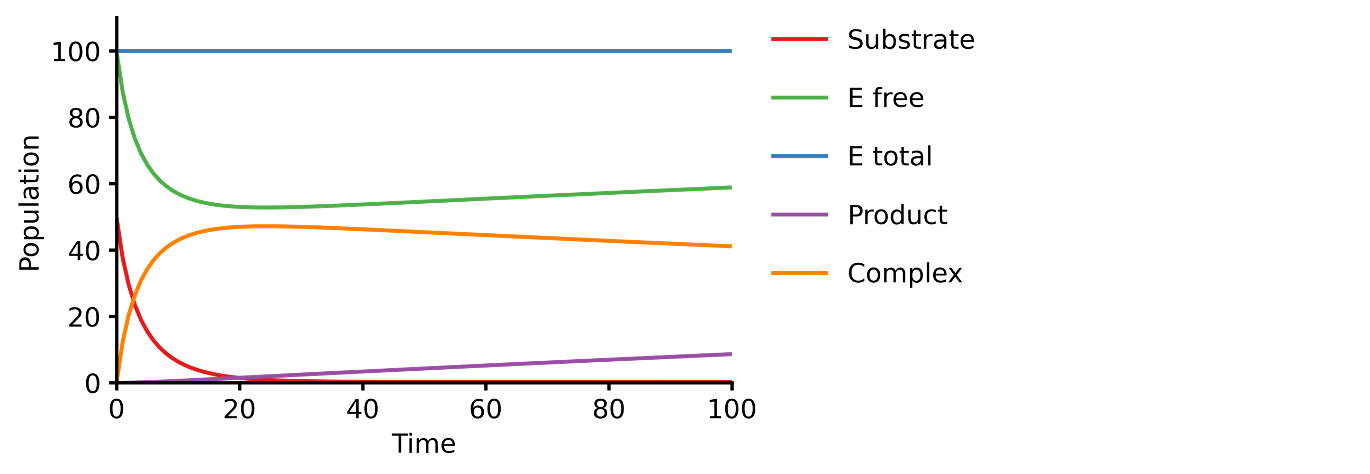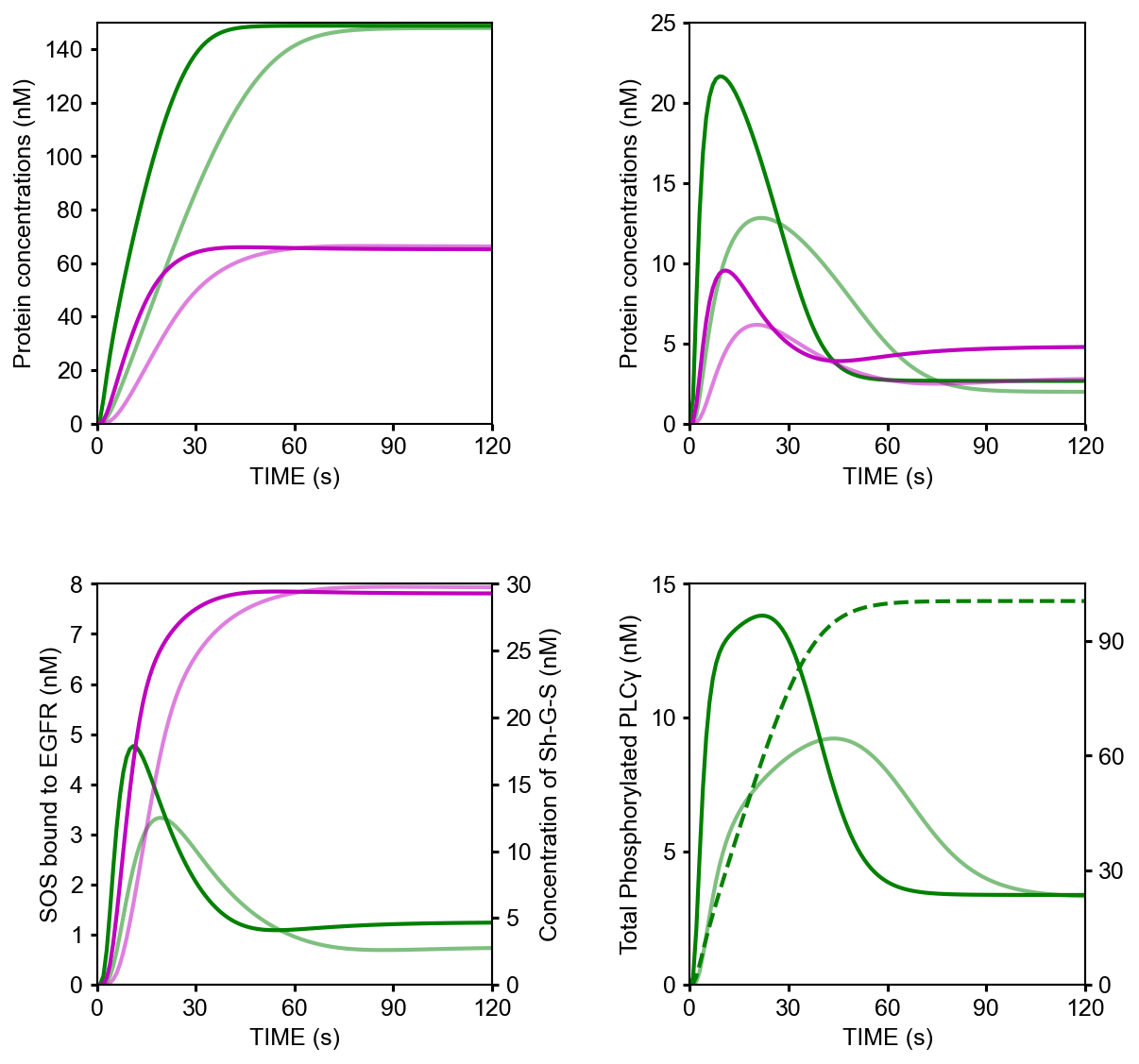# Model development

This section walk you through the creation of a mechanistic model from text.

## How to use

`pasmopy.Text2Model` is a useful class to build an ordinary differential equation (ODE) model from a text file describing biochemical systems.

The text file you need to prepare can be divided into three parts:

Note

To write a comment, simply put the hash mark # before your desired comment.

# This is a comment.

### Reaction layer

description | parameters | initial conditions

In the reaction layer, you need to describe biochemical reactions. Each reaction described in the line number i will be converted into ith rate equation. To specify parameters or initial conditions, you can put those information after `|`.

• If you don’t specify parameters/initial_conditions, they are initialized to 1 and 0, respectively, and the parameter values will be estimated from experimental data.

• If you want to set a parameter value to 1 and don’t want to estimate, you can add `const` prefix:

```# The Hill coefficient is fixed to 1.
TF transcribes mRNA | const n=1
```
• You can impose parameter constraints by specifying line number in the parameter section.

```1# Nucleocytoplasmic Shuttling of DUSP
2DUSPc translocates from the cytoplasm to the nucleus <--> DUSPn
3pDUSPc translocates from the cytoplasm to the nucleus <--> pDUSPn |2|
```

In the example above, you can assume that import and export rates were identical for DUSP (line 2) and pDUSP (line 3).

• If the amount of a model species should be held fixed (never consumed) during simulation, you can add `fixed` prefix:

```# [Ligand] will be held fixed to 10.0 during simulation
Ligand binds Receptor <--> LR | kf = 1e-6, kr = 1e-1 | fixed Ligand = 10.0
```

The available rules can be found at Available reaction rules (pasmopy.construction.reaction_rules).

You can also supply your own terminology in a reaction rule via:

```from pasmopy import Text2Model

# Supply "releases" to the reaction rule: "dissociate"
mm_kinetics = Text2Model("michaelis_menten.txt")
mm_kinetics.register_word({"dissociate": ["releases"]})
# Now you can use "releases" in your text, e.g., 'ES releases E and P'
mm_kinetics.convert()
```

### Observable layer (Prefix: `@obs`)

In the observable layer, you need to specify `biomass.observables`, which can correlate model simulations and experimental measurements. You can create an observable by using model parameters (`p`) and species (`u`). For example, if the total amount of SOS bound to EGFR should be the sum of RGS (EGFR-Grb2-SOS) and RShGS (EGFR-Shc-Grb2-SOS) complexes in your model, then you can write as follows:

```@obs Total_SOS_bound_to_EGFR: u[RGS] + u[RShGS]
```

### Simulation layer (Prefix: `@sim`)

In the simulation layer, you can set simulation conditions, e.g, the simulation time span, the initial concentration of model species, etc.

Example:

```@sim tspan: [0, 120]
@sim unperturbed: init[EGF] = 0
@sim condition EGF20nM: init[EGF] = 680
@sim condition EGF2nM: init[EGF] = 68
```
• tspan:

Two element vector `[t0, tf]` specifying the initial and final times.

• unperturbed (optional):

Description of the untreated condition to find the steady state.

• condition (optional):

Experimental conditions. Use `p` and `init` to modify model parameters and initial conditions, respectively.

## Examples

### Michaelis-Menten enzyme kinetics

This example shows you how to build a simple Michaelis-Menten two-step enzyme catalysis model with Pasmopy.

E + S ⇄ ES → E + P

An enzyme, E, binding to a substrate, S, to form a complex, ES, which in turn releases a product, P, regenerating the original enzyme.

1. Prepare a text file describing biochemical reactions (e.g., `michaelis_menten.txt`)

``` 1E binds S <--> ES | kf=0.003, kr=0.001 | E=100, S=50
2ES dissociates to E and P | kf=0.002, kr=0
3
4@obs Substrate: u[S]
5@obs E_free: u[E]
6@obs E_total: u[E] + u[ES]
7@obs Product: u[P]
8@obs Complex: u[ES]
9
10@sim tspan: [0, 100]
```
2. Convert the text into an executable model

```\$ python
```
```>>> from pasmopy import Text2Model
>>> description = Text2Model("michaelis_menten.txt")
>>> description.convert()
Model information
-----------------
2 reactions
4 species
4 parameters
```
3. Run simulation

```>>> from pasmopy import Model, run_simulation
>>> import michaelis_menten
>>> model = Model(michaelis_menten.__package__).create()
>>> run_simulation(model)
```### EGF signaling

Below is an example of Pasmopy in action to illustrate EGF signalling pathway.

Reference:

Kholodenko, B. N., Demin, O. V, Moehren, G. & Hoek, J. B. Quantification of short term signaling by the epidermal growth factor receptor. J. Biol. Chem. 274, 30169–30181 (1999). https://doi.org/10.1074/jbc.274.42.30169

1. Prepare a text describing EGF signaling in hepatocytes (`Kholodenko1999.txt`)

``` 1EGF binds EGFR <--> Ra | kf=0.003, kr=0.06 | EGFR=100
2Ra dimerizes <--> R2 | kf=0.01, kr=0.1
3R2 is phosphorylated <--> RP | kf=1, kr=0.01
4RP is dephosphorylated --> R2 | V=450, K=50
5RP binds PLCg <--> RPL | kf=0.06, kr=0.2 | PLCg=105
6RPL is phosphorylated <--> RPLP | kf=1, kr=0.05
7RPLP is dissociated into RP and PLCgP | kf=0.3, kr=0.006
8PLCgP is dephosphorylated --> PLCg | V=1, K=100
9RP binds Grb2 <--> RG | kf=0.003, kr=0.05 | Grb2=85
10RG binds SOS <--> RGS | kf=0.01, kr=0.06 | SOS=34
11RGS is dissociated into RP and GS | kf=0.03, kr=4.5e-3
12GS is dissociated into Grb2 and SOS | kf=1.5e-3, kr=1e-4
13RP binds Shc <--> RSh | kf=0.09, kr=0.6 | Shc=150
14RSh is phosphorylated <--> RShP | kf=6, kr=0.06
15RShP is dissociated into ShP and RP | kf=0.3, kr=9e-4
16ShP is dephosphorylated --> Shc | V=1.7, K=340
17RShP binds Grb2 <--> RShG | kf=0.003, kr=0.1
18RShG is dissociated into RP and ShG | kf=0.3, kr=9e-4
19RShG binds SOS <--> RShGS | kf=0.01, kr=2.14e-2
20RShGS is dissociated into ShGS and RP | kf=0.12, kr=2.4e-4
21ShP binds Grb2 <--> ShG | kf=0.003, kr=0.1
22ShG binds SOS <--> ShGS | kf=0.03, kr=0.064
23ShGS is dissociated into ShP and GS | kf=0.1, kr=0.021
24RShP binds GS <--> RShGS | kf=0.009, kr=4.29e-2
25PLCgP is translocated to cytoskeletal or membrane structures <--> PLCgP_I | kf=1, kr=0.03
26
27# observable layer
28@obs Total_phosphorylated_Shc: u[RShP] + u[RShG] + u[RShGS] + u[ShP] + u[ShG] + u[ShGS]
29@obs Total_Grb2_coprecipitated_with_Shc: u[RShG] + u[ShG] + u[RShGS] + u[ShGS]
30@obs Total_phosphorylated_Shc_bound_to_EGFR: u[RShP] + u[RShG] + u[RShGS]
31@obs Total_Grb2_bound_to_EGFR: u[RG] + u[RGS] + u[RShG] + u[RShGS]
32@obs Total_SOS_bound_to_EGFR: u[RGS] + u[RShGS]
33@obs ShGS_complex: u[ShGS]
34@obs Total_phosphorylated_PLCg: u[RPLP] + u[PLCgP]
35
36# simulation layer
37@sim tspan: [0, 120]
38@sim condition EGF20nM: init[EGF] = 680
39@sim condition EGF2nM: init[EGF] = 68
40@sim condition Absence_PLCgP_transloc: init[EGF] = 680; p[kf25] = 0; p[kr25] = 0
```
2. Convert the text into an executable model

```\$ python
```
```>>> from pasmopy import Text2Model
>>> description = Text2Model("Kholodenko_JBC_1999.txt")
>>> description.convert(show_restrictions=True)
Model information
-----------------
25 reactions
23 species
50 parameters

Thermodynamic restrictions
--------------------------
{9, 12, 10, 11}
{15, 18, 21, 17}
{18, 22, 20, 19}
{17, 24, 12, 19}
{23, 24, 20, 15}
{23, 12, 22, 21}
```
3. Run simulation

```>>> from pasmopy import Model, run_simulation
>>> import Kholodenko_JBC_1999
>>> model = Model(Kholodenko_JBC_1999.__package__).create()
>>> run_simulation(model)
```
4. Plot simulation results

```%matplotlib inline
import os
import matplotlib.pyplot as plt
import numpy as np

def plot_simulation_results(res):

plt.figure(figsize=(9, 9))
plt.rcParams['font.family'] = 'Arial'
plt.rcParams['font.size'] = 12
plt.rcParams['axes.linewidth'] = 1
plt.rcParams['lines.linewidth'] = 2

plt.subplot(2, 2, 1)  # ----------------------------------------------------
for obs_name, color in zip(
['Total_phosphorylated_Shc', 'Total_Grb2_coprecipitated_with_Shc'],
['g', 'm'],
):
obs_idx = model.observables.index(obs_name)
for j, condition in enumerate(['EGF20nM', 'EGF2nM']):
plt.plot(
model.problem.t,
res[obs_idx, j],
color=color,
alpha=0.5 if condition == 'EGF2nM' else None,
)
plt.xlim(0, 120)
plt.xticks([30*i for i in range(5)])
plt.ylim(0, 150)
plt.xlabel("TIME (s)")
plt.ylabel("Protein concentrations (nM)")

plt.subplot(2, 2, 2)  # ----------------------------------------------------
for obs_name, color in zip(
['Total_phosphorylated_Shc_bound_to_EGFR', 'Total_Grb2_bound_to_EGFR'],
['g', 'm'],
):
obs_idx = model.observables.index(obs_name)
for j, condition in enumerate(['EGF20nM', 'EGF2nM']):
plt.plot(
model.problem.t,
res[obs_idx, j],
color=color,
alpha=0.5 if condition == 'EGF2nM' else None,
)
plt.xlim(0, 120)
plt.xticks([30*i for i in range(5)])
plt.ylim(0, 25)
plt.xlabel("TIME (s)")
plt.ylabel("Protein concentrations (nM)")

ax1=plt.subplot(2, 2, 3)  # ------------------------------------------------
ax2 = ax1.twinx()
for j, condition in enumerate(['EGF20nM', 'EGF2nM']):
ax1.plot(
model.problem.t,
res[model.observables.index('Total_SOS_bound_to_EGFR'), j],
color='g',
alpha=0.5 if condition == 'EGF2nM' else None,
)
ax2.plot(
model.problem.t,
res[model.observables.index('ShGS_complex'), j],
color='m',
alpha=0.5 if condition == 'EGF2nM' else None,
)
ax1.set_xlim(0, 120)
ax1.set_xticks([30*i for i in range(5)])
ax1.set_xlabel("TIME (s)")
ax1.set_ylim(0, 8)
ax2.set_ylim(0, 30)
ax1.set_ylabel("SOS bound to EGFR (nM)")
ax2.set_ylabel("Concentration of Sh-G-S (nM)")

ax1=plt.subplot(2, 2, 4)  # ------------------------------------------------
ax2 = ax1.twinx()
obs_idx = model.observables.index('Total_phosphorylated_PLCg')
ax1.plot(
model.problem.t,
res[obs_idx, model.problem.conditions.index('EGF20nM')],
'g',
)
ax1.plot(
model.problem.t,
res[obs_idx, model.problem.conditions.index('EGF2nM')],
'g',
alpha=0.5,
)
ax2.plot(
model.problem.t,
res[obs_idx, model.problem.conditions.index('Absence_PLCgP_transloc')],
'g--',
)
ax1.set_xlim(0, 120)
ax1.set_xticks([30*i for i in range(5)])
ax1.set_ylim(0, 15)
ax1.set_yticks([5*i for i in range(4)])
ax1.set_xlabel("TIME (s)")
ax1.set_ylabel("Total Phosphorylated PLCγ (nM)")
ax2.set_ylim(0, 105)
ax2.set_yticks([30*i for i in range(4)])

plt.show()

if __name__ == '__main__':